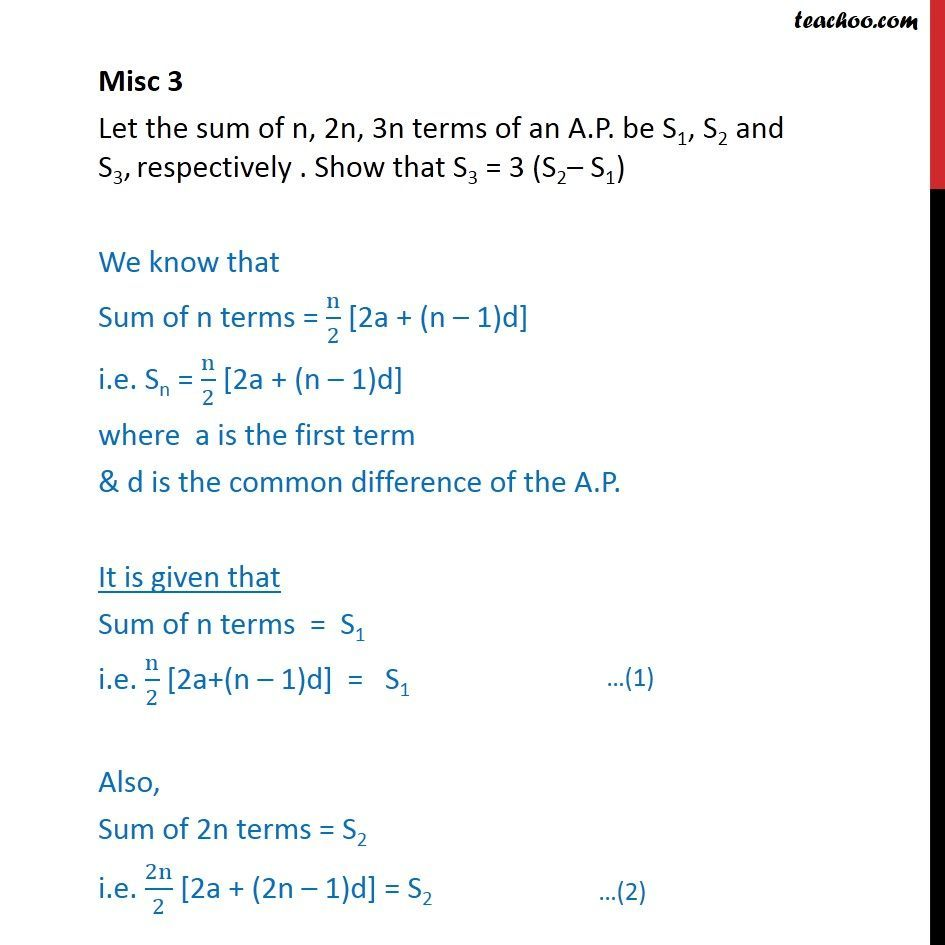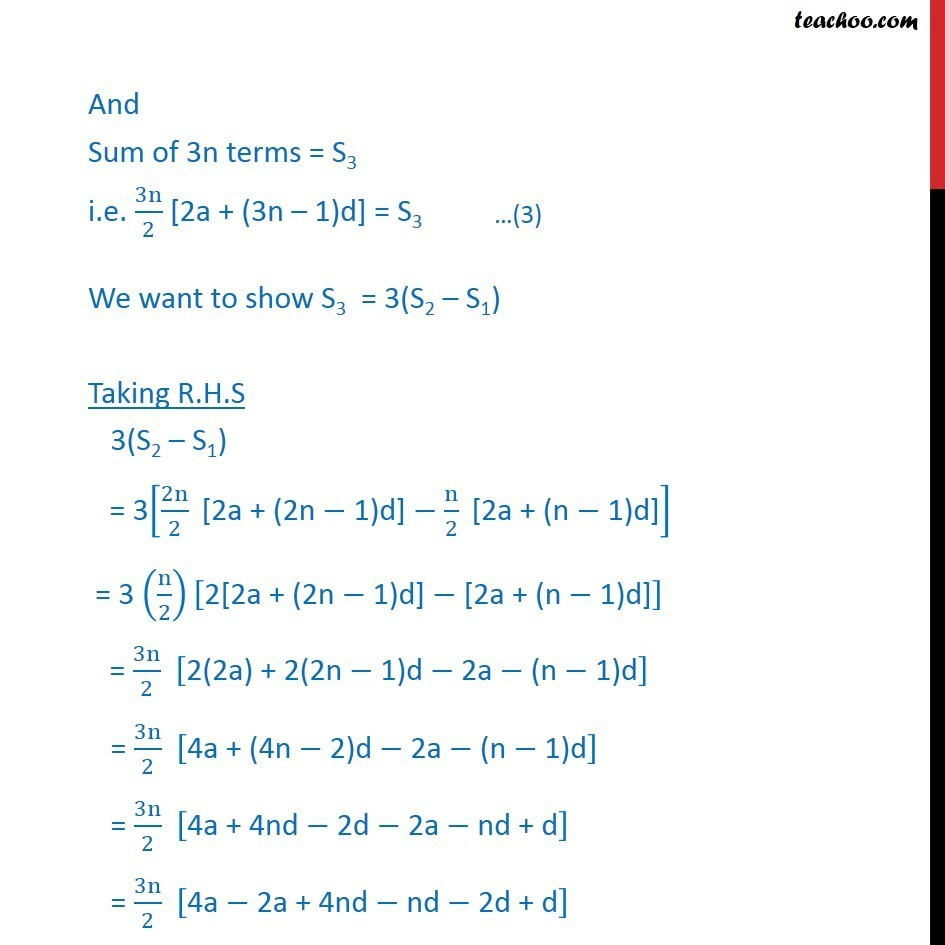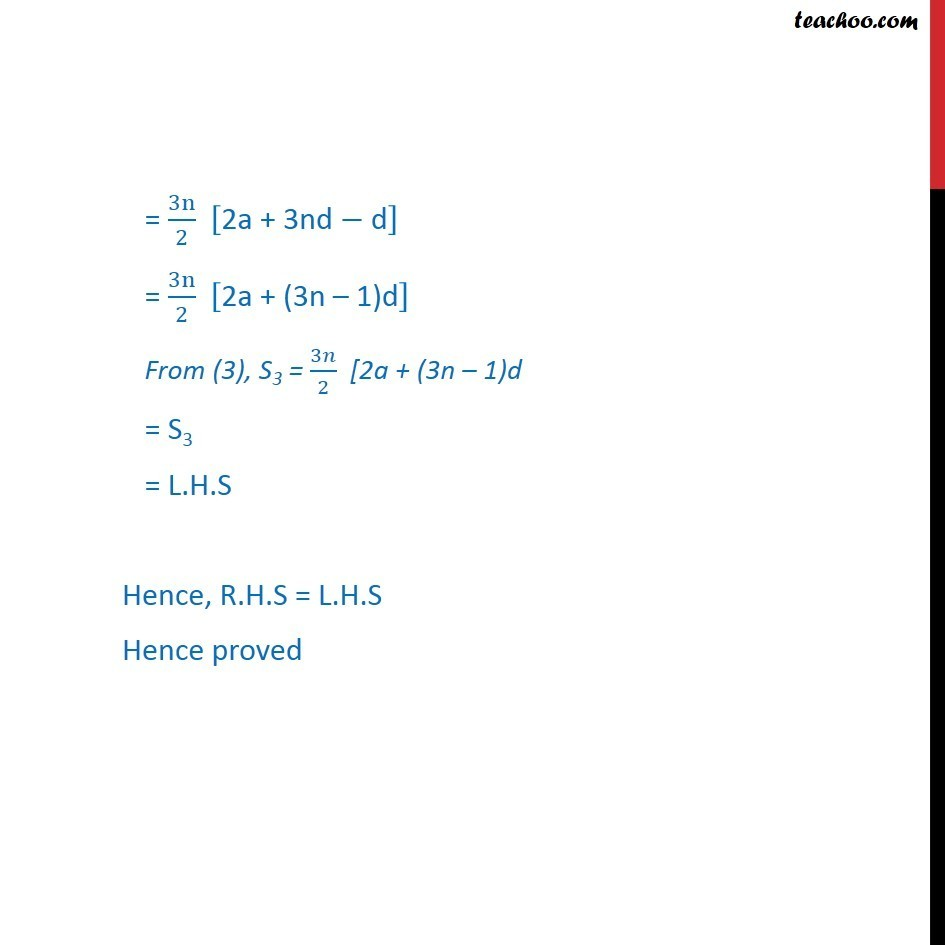Miscellaneous

Chapter 9 Class 11 Sequences and Series (Term 1)
Serial order wise983 students joined Teachoo Black. What are you waiting for?

### Transcript

Misc 3 Let the sum of n, 2n, 3n terms of an A.P. be S1, S2 and S3, respectively . Show that S3 = 3 (S2– S1) We know that Sum of n terms = n/2 [2a + (n – 1)d] i.e. Sn = n/2 [2a + (n – 1)d] where a is the first term & d is the common difference of the A.P. It is given that Sum of n terms = S1 i.e. n/2 [2a+(n – 1)d] = S1 Also, Sum of 2n terms = S2 i.e. 2n/2 [2a + (2n – 1)d] = S2 And Sum of 3n terms = S3 i.e. 3n/2 [2a + (3n – 1)d] = S3 We want to show S3 = 3(S2 – S1) Taking R.H.S 3(S2 – S1) = 3[2n/2 " [2a + (2n" −"1)d]" −n/2 " [2a + (n" −"1)d]" ] = 3 (n/2)["2[2a + (2n" −"1)d]" −"[2a + (n" −"1)d]" ] = 3n/2 ["2(2a) + 2(2n" −"1)d" −"2a" −"(n" −"1)d" ] = 3n/2 ["4a + (4n" −"2)d" −"2a" −"(n" −"1)d" ] = 3n/2 ["4a + 4nd" −"2d" −"2a" −"nd + d" ] = 3n/2 ["4a" −"2a + 4nd" −"nd" −"2d + d" ] = 3n/2 ["2a + 3nd" −"d" ] = 3n/2 ["2a + (3n – 1)d" ] From (3), S3 = 3𝑛/2 [2a + (3n – 1)d = S3 = L.H.S Hence, R.H.S = L.H.S Hence proved